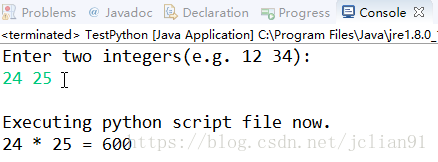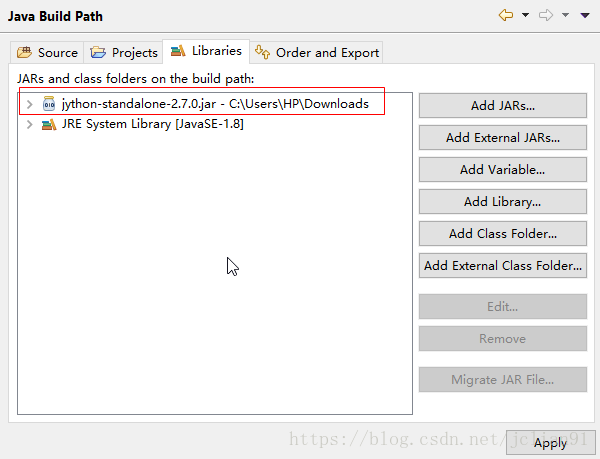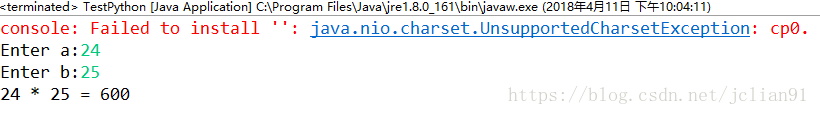## Java之调用Python代码 转载：https://mp.weixin.qq.com/s/cr8dXzwsQhtei9TfXwcMcA

想要在Java中调用Python代码，有以下两个办法：

• 直接通过Runtime进行调用

• 调用Jython

接下来，我们将分别介绍以上两种调用方法。
首先是直接通过Runtime调用Python代码。先写个Python代码文件test_argv.py，它位于D盘中，其完整代码如下：

import sysa = sys.argvb = sys.argvprint("%s * %s = %s"%(a,b,int(a)*int(b)))

package javaCode;import java.io.BufferedInputStream;import java.io.BufferedReader;import java.io.InputStreamReader;import java.util.Scanner;public class TestPython {    public static void main(String[] args) throws Exception {        Scanner input = new Scanner(System.in);        // 在同一行输入两个数字，用空格分开，作为传入Python代码的命令行参数        System.out.println("Enter two integers(e.g. 12 34): ");        String integers = input.nextLine();        String[] numbers = integers.split(" ");        // 定义传入Python脚本的命令行参数，将参数放入字符串数组里        String cmds = String.format("python D://test_argv.py %s %s",                                     numbers, numbers);        // 执行CMD命令        System.out.println("\nExecuting python script file now.");        Process pcs = Runtime.getRuntime().exec(cmds);        pcs.waitFor();        // 定义Python脚本的返回值        String result = null;        // 获取CMD的返回流        BufferedInputStream in = new BufferedInputStream(pcs.getInputStream());        // 字符流转换字节流        BufferedReader br = new BufferedReader(new InputStreamReader(in));        // 这里也可以输出文本日志        String lineStr = null;        while ((lineStr = br.readLine()) != null) {            result = lineStr;        }        // 关闭输入流        br.close();        in.close();        System.out.println(result);    }}程序输出结果

然后我们将演示如何使用Jython来实现类似上述程序的功能。Java Build Path中加入jython-standalone-2.7.0.jar

Java代码如下：

package javaCode;import org.python.util.PythonInterpreter;public class TestPython {    public static void main(String args[]) {        PythonInterpreter interpreter = new PythonInterpreter();        interpreter.exec("import sys ");        interpreter.exec("a = input('Enter a:')");        interpreter.exec("b = input('Enter b:')");        interpreter.exec("print('%s * %s = %s' %(a, b, a*b))");    }}运行结果

至此，我们成功地用以上两种方法在Java中调用了Python代码

posted on 2020-07-21 18:32  我和你并没有不同  阅读(28)  评论(0编辑  收藏

• 随笔 - 355
• 文章 - 0
• 评论 - 6